# Using DATE function in Excel - formula examples to calculate dates

When it comes to calculating dates in Excel, DATE is the most essential function to understand. As you probably know, Excel does not keep the year, month and day for a date, nor does it explicitly store weekday information in a cell. Instead, Microsoft Excel stores dates as serial numbers and this is the main source of confusion.

Not all Excel date functions can recognize dates entered as text values, therefore it's not recommended to supply dates directly in calculations. Instead, you should use the DATE function to get a serial number representing the date, the number that Excel understands and can operate on.

## Excel DATE function

The Excel DATE function returns the serial number that represents a certain date. It has the following arguments:

DATE(year, month, day)

Where:

Year - represents the year of the date.

Month - an integer representing the month of the year, from 1 (January) to 12 (December).

Day - an integer corresponding to the day of the month, from 1 to 31.

The DATE function is available in all versions of Excel 365 - Excel 2007.

### DATE function - tips and notes

The DATE syntax looks crystal clear and straightforward on the surface. In practice, there may be some unobvious pitfalls that the below tips will help you avoid.

#### Year

Excel interprets the year argument according to the date system set up on your computer. By default, Microsoft Excel for Windows uses the 1900 system where January 1, 1900 is represented by the serial number 1. For more details, please see Excel date format.

• If the year argument is between 1900 and 9999 inclusive, Excel uses exactly the value you supplied to create a date. For example, DATE(2015, 12, 31) returns December 31, 2015.
• If the year argument is between 0 and 1899 inclusive, Excel adds the specified number to 1900. For example, DATE(100, 12, 31) returns December 31, 2000 (1900 + 100).
• If the year argument is less than 0 or greater than 9999, a DATE formula will return the #NUM! error.

Tip. To avoid confusion, always supply four-digit years. For example, if you input "01" or "1" in the year argument, your DATE formula will return the year of 1901.

#### Month

• If the month argument is greater than 12, Excel adds that number to the first month in the specified year. For example, DATE(2015, 15, 5) returns the serial number representing March 1, 2016 (January 5, 2015 plus 15 months).
• If the month argument is less than 1 (zero or negative value), Excel subtracts the magnitude of that number of months, plus 1, from the first month in the specified year. For example, DATE(2015, -5, 1) returns the serial number representing July 1, 2014 (January 1, 2015 minus 6 months).

#### Day

As well as month, the day argument can be supplied as a positive and negative number, and Excel calculates its value based on the same principles as described above.

Tip. At first sight, supplying negative values in the month or day argument of the Excel DATE function may seem absurd, but in practice it may turn out quite useful, for example in the complex formula that converts a week number to a date.

## Excel DATE formula examples

Below you will find a few examples of using DATE formulas in Excel beginning with the simplest ones.

### Example 1. DATE formula to return a serial number for a date

This is the most obvious use of the DATE function in Excel.

For example, to return a serial number corresponding to 20-May-2015, use this formula:

`=DATE(2015, 5, 20)`

Instead of specifying the values representing the year, month and day directly in a formula, you can have some or all arguments driven by of other Excel date functions. For instance, combine the YEAR and TODAY to get a serial number for the first day of the current year.

`=DATE(YEAR(TODAY()), 1, 1)`

And this formula outputs a serial number for the first day of the current month in the current year:

`=DATE(YEAR(TODAY()), MONTH(TODAY(), 1)`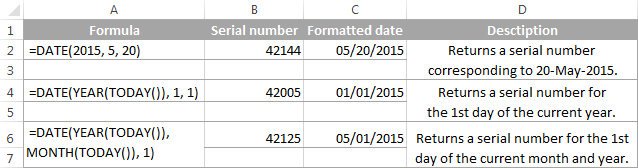Tip. To display a date rather than a serial number, apply the desired Date format to the formula cell.

### Example 2. How to return a date based on values in other cells

The DATE function is very helpful for calculating dates where the year, month, and day values are stored in other cells.

For example, to find the serial number for the date, taking the values in cells A2, A3 and A4 as the year, month and day arguments, respectively, the formula is:

`=DATE(A2, A3, A4)`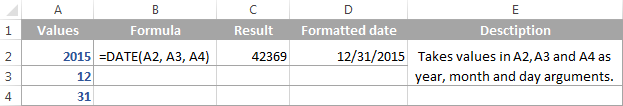### Example 3. DATE formula to convert a string or number to a date

Another scenario when the Excel DATE function proves useful is when the dates are stored in the format that Microsoft Excel does not recognize, for instance DDMMYYYY. In this case, you can use DATE in liaison with other functions to convert a date stored as a numeric string or number into a date:

`=DATE(RIGHT(A2,4), MID(A2,3,2), LEFT(A2,2))`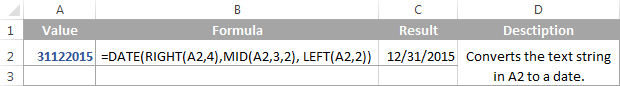### Example 4. Adding and subtracting dates in Excel

As already mentioned, Microsoft Excel stores dates as serial numbers and operates on those numbers in formulas and calculations. That is why when you want to add or subtract some days to/from a given date, you need to convert that date to a serial number first by using the Excel DATE function. For example:

• Adding days to a date:`=DATE(2015, 5, 20) + 15`The formula adds 15 days to May 20, 2015 and returns June 4, 2015.
• Subtracting days from a date:`=DATE(2015, 5, 20) - 15`The result of the above formula is May 5, 2015, which is May 20, 2015 minus 15 days.
• To subtract a date from today's date:`=TODAY()-DATE(2015,5,20)`The formula calculates how many days are between the current date and some other date that you specify.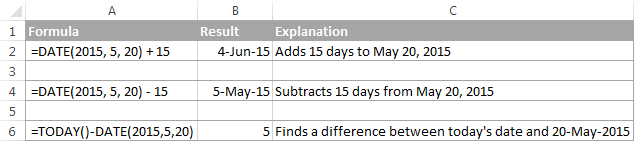If you are adding or subtracting two dates that are stored in some cells, then the formula is as simple as =A1+B1 or A1-B1, respectively.

And here are a few more examples where Excel DATE is used in combination with other functions in more complex formulas:

## Using Excel DATE formulas in conditional formatting

In case you want not only to calculate but also highlight dates in your Excel worksheets, then create conditional formatting rules based on DATE formulas.

Supposing you have a list of dates in column A and you want to shade dates that occurred earlier than 1-May-2015 in orange and those that occur after 31-May-2015 in green.

The DATE formulas you want are as follows:

Orange: `=\$A2<DATE(2015, 5, 1)` - highlights dates less than 1-May-2015

Green: `=\$A2>DATE(2015, 5, 31)` - highlights dates greater than 31-May-2015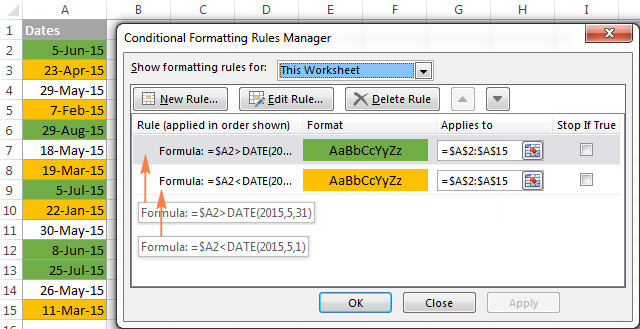For the detailed steps and more formula examples, please see How to conditionally format dates in Excel.

## How to make date formulas in Excel with Date & Time Wizard

Though DATE is the main function to work with dates in Excel, a handful of other functions are available to tackle more specific tasks. You can find the links to in-depth tutorials at the end of this article.

Meanwhile, I'd like to present you our Date & Time Wizard - a quick and easy way to calculate dates in Excel. The beauty of this tool is that outputs the results as formulas, not values. Thus you have a kind of 'two birds, one stone' opportunity - get the result faster and learn Excel date functions along the way :)

The wizard can perform the following calculations:

• Add years, months, weeks, days, hours, minutes and seconds to the specified date.
• Subtract years, months, weeks, days, hours, minutes and seconds from the specified date.
• Calculate the difference between two dates.
• Get age from the birthdate.

For example, here's how you can add 4 different units in cells B3:E3 to the date in A4. The formula in B4 is built in real-time as you change the conditions: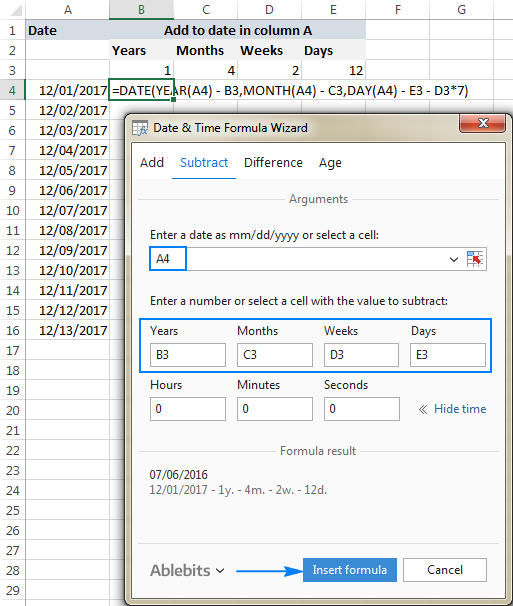If you are curious to explore other capabilities of the wizard, feel free to download an evaluation version of the Ultimate Suite below which includes this as well as 60 more time saving add-ins for Excel.

I thank you for reading and hope to see you on our blog next week!

## Excel dates tutorials:

1. HELP! I just want to know if Excel has a way to make a column with each cell representing Week 1 - then the dates of that week for 2017. For example the cell would read: Week 1 - January 1-7 (or better if it was just work week, Jan 2-6). Can this be done??? Please help me.

2. Hi There

I am trying to calculate the number of days between two entries. I am using the following formula.

=IF(F4='''',NETWORKDAYS(E4,TODAY()),NETWORKDAYS(E4,F4))

The formula works but when I use a blank cell (F4) the formula stops working.

3. hi i need to formula this.

i have a start date lets say:

start date : 1/9/2016
i applied to be on leave for months

what is the end date should be?

4. I am working on a training matrix. I have a column with first aid. If it is past current date it should go red. The first aid is valid for 3 years I need it to go yellow 3 months before it is set to expire using date in column and not current date. So it it is valid it is green, if it is past due it is red and if it is 3 months before it turns red or past due it is yellow to warn me to get them trained. Any suggestions.

Second column is training that is due annually. So red if past due, green if ok and yellow again three months before date in column's one year mark as a warning to get them re-certified.

Can anyone help with these formulas. Any date formula tips for training matrix are welcome. Thanks

5. I want to change this date format 1/1/2016 12:00:00 AM
into fiscal years, 15-16, can anyone help.

6. I'm trying to figure out when an employee is eligible for enrolment in our pension plan. I have the following:
C3=start date with company
D3=eligibility date=6 months after start date
The plan didn't become effective until July 1, 2013 so I'm looking for a formula that says if C3 is less than or equal to July 1, 2013 then use July 1, 2013, if not then use C3 plus 6 months.
Any help would be fantastic.

7. If I have a start date and end date are as per Hijiri calendar, to check if it is expired or not so how i can apply this =If(today()>=A1,"Expired","Not Expired")

i tried below function but nothing changes

ype a date in Gregorian format and have Excel interpret it as Hijri date:
Go to Custom Format and enter B2dd/mm/yyyy.
The date will be displayed as Hijri date.

8. hello i need to get some formulae to calculate data that falls under particular month in a sheet with different columns of date for eg.
we got columns as
customer address date1 date2 date3 date4
gaurav india 1/4/15 24/5/15 21/7/15 19/8/15
like this upto 12 date columns and there is no limit for the no. of rows.
so i need some help if i want to get data for the month of may, it shows me this customer detail. really appreciate your response…. thanks

9. Hello,
You truly are incredible. I need to determine if a date in a range exists. If it exists then I would like it entered it into another cell. Is there a simple way to do this?
Thank you!

10. Hello! I have a project at work where a deficiency needs to be completed by 7 days after the original deadline. I am looking for a formula where a column is turned red after 7 days-does this exist?? :s

11. Hi
Please help. I'm subtracting two dates to calculate number of holidays taken. The two dates are in two separate columns with answer in the third column e.g.
A12 Date leave started
B12 Date leave ended
C12 Formula answer with No of Days Leave Taken.

The problem arises when someone takes 1 day's leave; my formula says 0 Days. For example:
A12 Date leave started 21/05/2016
B12 Date leave ended 21/05/2016
C12 Formula answer with No of Days Leave Taken. Result is 0 days. I want it to say 1 day's leave taken.
Thank you

12. how do I find a date after some days of a specific date.
for example,let installation date of a product is 5/15/2013
and the life span of that product is 1500 days.how do I find out the replacement date of that product.is there any excel function for that.?

13. How to calculate the date of retirement of an employee after completion of 60 years.

14. Hi Ablebits,

I need help on dates formatting. I have a list of dates as far as 2014 until today. I would like to highlight dates that are 30 days from the date in the cell, 60 days and 90 days. I've been Google-ing answers for days, but to no success. Please help.

15. hai

can somebody help how to make tabel for this appoitment date

example;-

date - MAIN DATE { 29-aug-2016(monday)}

week month
1. 05 sept 2016 26 sept 2016
2. 12 sept 2016 31 oct 2016
3. 19 sept 2016 28 nov 2016
4. 26 sept 2016 26 dec 2016
5. 03 oct 2016 30 jan 2017
6. 10 sept 2016 27 feb 2017

the week date i can do but the date for month i can't do
b'coz the month date must +/- 3 day from main date (29 AUG 2016)...

can somebody help me thanks..

16. In answer to Stacie (83), I think

=min(date1,date2)-30 should work if you format the cell as a date.

I don't know if that will meet your needs, but the math seems to be good.

HTH!

Tom

17. I'm sorry if this has been covered previously!

I am calculating the number of days between two dates, using one column for the start date and a second column for the end date. the third column is the number of days between the two dates. All is well until the dates span the February/March period during leap year. In that case, the calculation is incorrect by a day. Is this a bug in the Excel "DAYS" function? Is there a way around it?

Thanks!

Tom

18. Hello,

I am trying to find a formula to calculate the following:

Look at the date in cell A2
Look at the date in cell A3
Figure out which is the earliest date
Then subtract 4 weeks (or 30 days) from the earliest date in either cell.

Is this possible?

Thanks!

19. Hi , i am trying to convert a cell with the following text 1/18/2016 10:00:00 am to 2016/1/18 . but i cannot seem to make it work with datevalue. Kindly help ..

20. Hello I am trying to identify due dates for training. We have several different trainings that are required, and they all have different frequencies (i.e. annual, bi-annual, semi-annual, etc). Is there a way to identify when the next due date would be if I have one column that has the date the training was taken, a column showing frequency (annual, semi-annual, etc.) and then a column with new due date?

• Hello Tania,

To help you better, we need a sample table with your data in Excel and the result you want to get.

21. I am creating dropdown menu for the start date and end date of all projects, but would like to reflect in the column. How do I proceed? Example 12/23/2015 - 12/22/2017.

22. I need to create a formula that calculates the number of sick days an employee gets from hire date (1 day at 6 mos, 2 days at 1 yr, 3 days at 2 yrs, 4 days at 3+ yrs) based upon the current date at any given time [i.e. TODAY()]. Can you please help me? Thank you!!!

23. I am trying to find a formula that will take D28(which is a date) and then will compare to a list of dates(US Holidays that the list has a defined named as Holidays_US) and if it is in that list then I want to return D28 plus a day and if it isn't in that list then I want to return D28+365. I can't figure it out please help if you are able to. Thank you!

24. I want subtract two date but some cell getting blank that time i wants if cells are black their getting today values

25. I want these remain dates in this row & Column, Plz help me how I can put the formulas
1-Aug-16 10-Aug-16 11-Aug-16 31-Aug-16
2-Aug-16 11-Aug-16 12-Aug-16 31-Aug-16
3-Aug-16 12-Aug-16
4-Aug-16 13-Aug-16
5-Aug-16 14-Aug-16
6-Aug-16 15-Aug-16
7-Aug-16 16-Aug-16
8-Aug-16 17-Aug-16
9-Aug-16 18-Aug-16
10-Aug-16 19-Aug-16

26. Im trying to calculate a prison sentence of 24months(2years) commencing today 8/8/2016. Release date is 8/8/2018, however a remission of one third(1/3) was deducted from 24 months. Can any excel elite calculate the new release date?

Sentence date: 8/8/2016
period sentence: 24 months
Release date: 8/8/2018
Remission: 1/3
New release date??????

27. I am trying to calculate an end date using a start date and the number of weeks. EX start date 9/5/2016 need to go out 52 weeks = what would end date be? Is this type of calculation possible or do I need to convert Weeks to days?

28. or have a formula 031214H August 2016 convert to 08/03/2016... thanks you

• Hi BERNARD,

Try this formula:
=DATEVALUE(LEFT(A2,2)&"-"&MID(A2,SEARCH(" ",A2)+1,SEARCH(" ",A2,SEARCH(" ",A2)+1)-SEARCH(" ",A2)-1)&"-"&RIGHT(A2,4))

Where A2 is the cell containing the string 031214H August 2016. Also, be sure to apply the desired date format to the formula cell.

29. good day, any one to help me to have a formula 031214H August 2016 convert to 08/03/2016... thanks you

30. Hi there. Great page but hoping you can answer my query?

In column A I have a date in format DD/MM/YYYY and want to convert this to be displayed in the MM/DD/YYYY format (or the serial number relating to the date) in column B. I have used Text To Columns to do this but this will not update column B if the value in column A is changed or if a new date is added in the next row down.

Is there any way I can do this using the DATE, DATEVALUE or any other functions?

Thanks :)

• Hi Mike,

I can suggest the following 2 ways:

1. =TEXT(A1, "mm/dd/yyyy") - the result will be a text string, but not a date.

2. Use this simple formula =A1, and then apply the desired format to the 2nd column (select the column, right click, click Format Cells, select Custom under Category, and type mm/dd/yyyy in the Type box). The resut will be a fully-functional date in the desired format.

31. I want to change the date format 7/30/2016 in dd-mm-yyy and it should be 30-07-2016. Please help

• Hi Aman,

Simply press Ctrl+1 to open the Format Cells dialog, select Custom under Category, and type the following format in the Type box: dd-mm-yyyy

For the detailed instructions, please see How to create a custom date format in Excel.

32. Hello, can anyone help me what is excel formula if the starting date will tell it is overdue in: equal or less than 3 months, greater than 3 months, greater than or equal to 6 months?

33. Hello,

I have two dates in two different cells (A1 = 4/12/1993 and B1 = 04/05/1993) and i want to verify if they fall with the same quarter. If two dates are within the same quarter, the data "passed" if outside of 89 days, it fails...

34. I have found some valuable info on this great site, but when I try to put what I find into use I keep getting error messages. I'm guessing due to the complexity and my lack of knowledge it's simply not going to work just piecing together the formulae I need from the info I find here.
I need a formula to work out compliance to a medical standard, the cells are as follows;
C1 is date of birth
F1 is date of last medical
I need to return a yes or no in G1.
If the subject is over 35 years old the date in F1 has to be less than 5 years ago to return yes. If the subject is under 35 years old F1 has to be within the last 10 years to return a yes value.
I have been going round in circles with not much luck in creating the right formula.

• My only working solution is the formula below, but this does not account for the age of the subject. I can't get the link to the age to work correctly.
=IF(DATEDIF(F1, TODAY(), "y")<5,"YES","NO")

• Hi Elyes,

If my understanding of the task is correct, the following formula should work a treat:

=IF(OR(AND(DATEDIF(C1, TODAY(), "y")>=35, DATEDIF(F1, TODAY(), "y")<5), AND(DATEDIF(C1, TODAY(), "y")<35, DATEDIF(F1, TODAY(), "y")<10)), "yes", "no")

• Works brilliantly! Exactly what I was after. Many thanks.

35. i want to calculate date with the numbers then want the answer in date.
ex. date is 27/06/2016 then i add 08 in the other sell i want the ans in date format like 05/07/2016. plz help me

36. 21/01/2016 i want to twenty first january two thousand sixteen

37. Hi, I need a formula for dates.
For example,
11/1/15 through 2/1/16
11/1/15 through 3/1/16....etc
I need it where the first date does not change but the latter date changes. When I insert 11/1/15 - 2/1/16, I believe Excel takes the "through" as a subtracting sign. How do I fix this?
Thank you!

38. Hello,
I have this bi-weekly payroll start and end date.
I need a formula to calculate number of pays if I select a range from start and end date.
for example I want to see number of pays from start date 5/23/16 to 8/14/16 end days.

Start Date End Date
12/21/2015 1/3/2016
1/4/2016 1/17/2016
1/18/2016 1/31/2016
2/1/2016 2/14/2016
2/15/2016 2/28/2016
2/29/2016 3/13/2016
3/14/2016 3/27/2016
3/28/2016 4/10/2016
4/11/2016 4/24/2016
4/25/2016 5/8/2016
5/9/2016 5/22/2016
5/23/2016 6/5/2016
6/6/2016 6/19/2016
6/20/2016 7/3/2016
7/4/2016 7/17/2016
7/18/2016 7/31/2016
8/1/2016 8/14/2016
8/15/2016 8/28/2016
8/29/2016 9/11/2016
9/12/2016 9/25/2016
9/26/2016 10/9/2016
10/10/2016 10/23/2016
10/24/2016 11/6/2016
11/7/2016 11/20/2016
11/21/2016 12/4/2016
12/5/2016 12/18/2016

39. it is very good example to adding the formulas in Excel sheets and very good site to learn about excel.

40. Hi there

I have a sheet that we use for targets. Now if you pick a month it should run from the 11th to the 10th of the following month. My formula works fine but when a month has 30 days or 29 or 28 days it will run till the 12th or 11th instead of stopping at the 10th. How do I limit it to end till the 10th of each month.

41. hi ld like to ask what formula should I use for this. in E2 I have 01/01/2016. I want to create a formula so it will be converted to January in F2. what should I use?

• Hi Kit,

You can do this in 2 ways:

1. In F2, enter the formula =E2, and then apply the custom date format: mmmm
You can find the detailed explanation about custom formatting here: How to create a custom date format in Excel.

2. Use this formula: =TEXT(E2, "mmmm")

The difference between the above approaches is as follows:

1. Changes only the visual representation but the underlying value in F2 is the full date and it can be used in other calculations.

2. The value in F2 is a text string and not a date.

• OMG! You are amazingly super smart. The 2nd option worked for my need and report :). I really appreciate your help. you are AWESOME!

42. HI there,

I am currently creating a schedule where I want to go back 1 week -10 daysfrom a numerical date in a cell, but within that one week-10 days I want excel to choose the wednesday furthest back, how would I go about doing that.

Say my due date is September 1, 2016, going back 10 days will take me back to August 22 (monday). I want the cell to compute Wednesday, August 24. I have different dates to set up so it'll never be consistent like 5 days before etc. and I don't want to have to change for each instance.

Any help would be greatly appreciated!

43. hi,

i need some help/tip in calculating difference between two dates in one cell. i also try datedif formula but in office excel 2007 is don't exists. i am also look for help for converting 'ddmmyyyy'(7121985/31052005) in 'dd-mmm-yy'(07-Dec-85/31-Mar-05). I required all this for excel sheet 2007. Please help me.../////

44. Hii

equation for showing a particular date for a dategroup(means a week or a countious 5 days, workdays etc)

eg:01-05-2016 to 05-05-2016 is shown in a cell as 01-05-2016.

45. Hi,

I hope you can help me with my problem, i have a Received date and Fixed date in Cell A1 and B1, i need to calculate if A1 starts at <or=8:00 AM to 4:00 PM,maybe we can put it in C1 as Yes and for No. Then another calculation is closing Date if all Yes in C1 is closed at 5:00 PM, in No Calculate if closed within 24 hours. This except Sat and Sun.

Regards,
Dennis

46. I would like the return value of the cell to be the start of the current year if an employee started prior to the year starting and if they started within the year to return their hire date. Can you assist?

47. hello
How to calculate Total Exp( Sample Format : 10 Years 6 Months & 12 Days)
When i'm using DATEDIF() function it gives only year
please give me the idea how i can get Sample Format : 10 Years 6 Months & 12 Days

• Hello Anusha,

You can use the following formula:

=DATEDIF(A2, B2, "y") &" years " &DATEDIF(A2, B2, "ym") &" months & " &DATEDIF(A2, B2, "md") &" days"

Where A2 is the start date and B2 is the end date.

48. I'd like to know if this is possible.
I'm trying to get how many days are my computers hired in certain months.
Say, 1 computer is hired from June 10 to July 20, 2016.
Is their a turnaround/ formula on how to get the days hired in june and july in separate columns.
So for june I get 20 days hired, and july 20 days hired.
thanks!

• Dear Giovanni,

(1)How is your data organised? Are the start dates and end dates in 2 columns? Is the name or ID of the computer mentioned in another column? Can you send the details of the data in brief?

(2) In the period 10 June to 20 July, June has 21 days. Is the first day to be ignored?

When posting a question, please be very clear and concise.

Vijaykumar Shetye, Goa, India

49. Hi,

Thank you for giving such wonderful tips, i want to calculate daily productivity on excel like column A date column B references of accounts attended, how i can calculate number of accounts attended on a particular date.

Regards,

• Dear Muzzamal Azeem,

Use this array formula is column E
=SUMPRODUCT(IF(\$A\$1:\$A\$100=D1,1,0),IF(LEN(\$B\$1:\$B\$100)>=1,1,0))
Array formulas are entered with Control+Shift+Enter instead of Enter.

List of dates is in column D, and your data is in column A to B, as you have mentioned in your post.

A B C D E
1 01/01/2016 A/C 01-Jan 2
2 01/01/2016 A/C 02-Jan 4
3 01/01/2016 03-Jan 4
4 02/01/2016 04-Jan 5
5 02/01/2016 A/C 05-Jan 3

Vijaykumar Shetye, Goa, India

50. please help me how to calculate the days from 15-5-2016 to 26-5-2016 excluding holidays falling in the first 5 days from 15-5-2016 to 19-5-2016

• Dear Ravish,
Use the below formula
=NETWORKDAYS(A1,A2,B1:B100)

Cell references are as follows
Start date in cell A1,
End date in cell A2,
List of holidays in cells B1 to B100.
Change the cell references as required.

Vijaykumar Shetye, Goa, India